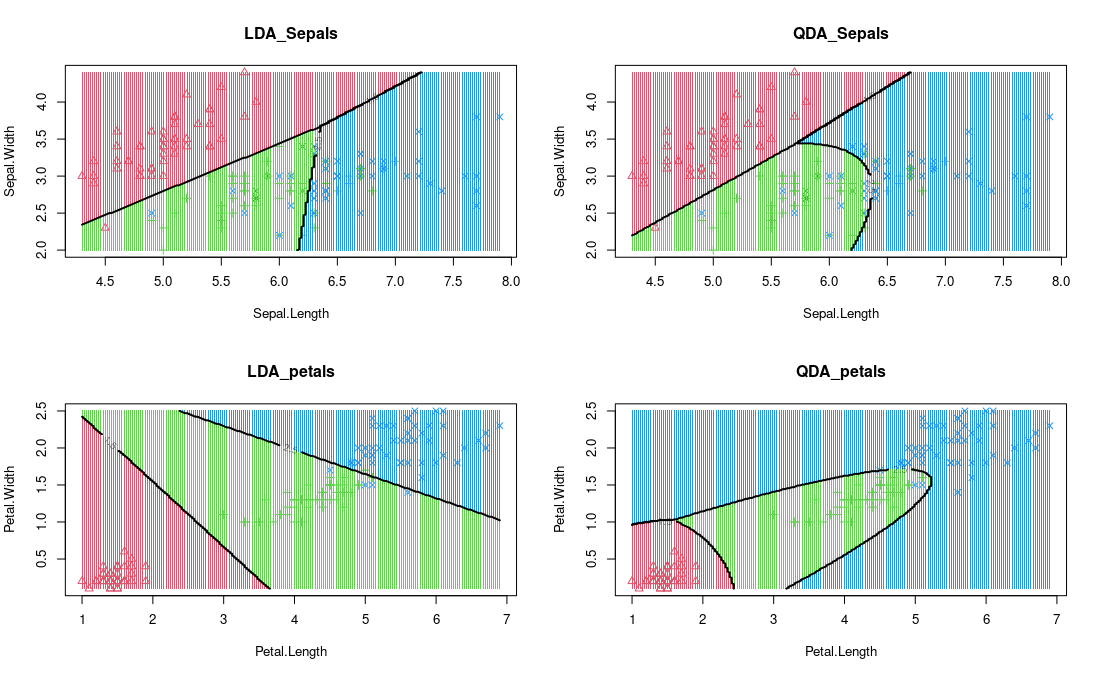Related Articles

• Last Updated : 18 Jul, 2021

### Linear Discriminant Analysis

Now, Let’s consider a classification problem represented by a Bayes Probability distribution P(Y=k | X=x), LDA does it differently by trying to model the distribution of X given the predictors class (I.e. the value of Y) P(X=x| Y=k):In LDA, we assume that P(X | Y=k) can be estimated to the multivariate Normal distribution that is given by following equation:where,and P(Y=k) =\pi_k. Now, we try to write the above equation with the assumptions:Now, we take log both sides and maximizing the equation, we get the decision boundary:For two classes, the decision boundary is a linear function of x where both classes give equal value, this linear function is given as:For multi-class (K>2), we need to estimate the pK means, pK variance, K prior proportions and. Now, we discuss in more detail about Quadratic Discriminant Analaysis.

Quadratic discriminant analysis is quite similar to Linear discriminant analysis except we relaxed the assumption that the mean and covariance of all the classes were equal. Therefore, we required to calculate it separately.

Now, for each of the class y the covariance matrix is given by:By adding the following term and solving (taking log both side and ). The quadratic Discriminant function is given by:### Implementation

• In this implementation, we will be using R and MASS library to plot the decision boundary of Linear Discriminant Analysis and Quadratic Discriminant Analysis. For this, we will use iris dataset:

## R

 # import librarieslibrary(caret)library(MASS)library(tidyverse)  # Code to plot decision plotdecision_boundary = function(model, data,vars, resolution = 200,...) {  class='Species'  labels_var = data[,class]  k = length(unique(labels_var))  # For sepals  if (vars == 'sepal'){  data = data %>% select(Sepal.Length, Sepal.Width)  }  else{  data = data %>% select(Petal.Length, Petal.Width)  }          # plot with color labels  int_labels = as.integer(labels_var)  plot(data, col = int_labels+1L, pch = int_labels+1L, ...)      # make grid  r = sapply(data, range, na.rm = TRUE)  xs = seq(r[1,1], r[2,1], length.out = resolution)  ys = seq(r[1,2], r[2,2], length.out = resolution)  dfs = cbind(rep(xs, each=resolution), rep(ys, time = resolution))      colnames(dfs) = colnames(r)  dfs = as.data.frame(dfs)      p = predict(model, dfs, type ='class' )  p = as.factor(p\$class)        points(dfs, col = as.integer(p)+1L, pch = ".")      mats = matrix(as.integer(p), nrow = resolution, byrow = TRUE)  contour(xs, ys, mats, add = TRUE, lwd = 2, levels = (1:(k-1))+.5)      invisible(mats)}  par(mfrow=c(2,2))# run the linear disciminant analysis and plot the decision boundary with Sepals variablemodel = lda(Species ~ Sepal.Length + Sepal.Width, data=iris)lda_sepals = decision_boundary(model, iris, vars= 'sepal' , main = "LDA_Sepals")  # run the quadratic disciminant analysis and plot the decision boundary with Sepals variablemodel_qda = qda(Species ~ Sepal.Length + Sepal.Width, data=iris)qda_sepals = decision_boundary(model_qda, iris, vars= 'sepal', main = "QDA_Sepals")  # run the linear disciminant analysis and plot the decision boundary with Petals variablemodel = lda(Species ~ Petal.Length + Petal.Width, data=iris)lda_petal =decision_boundary(model, iris, vars='petal', main = "LDA_petals")  # run the quadratic disciminant analysis and plot the decision boundary with Petals variablemodel_qda = qda(Species ~ Petal.Length + Petal.Width, data=iris)qda_petal =decision_boundary(model_qda, iris, vars='petal', main = "QDA_petals")LDA and QDA visualization

## References:

Attention reader! Don’t stop learning now. Get hold of all the important Machine Learning Concepts with the Machine Learning Foundation Course at a student-friendly price and become industry ready.

My Personal Notes arrow_drop_up